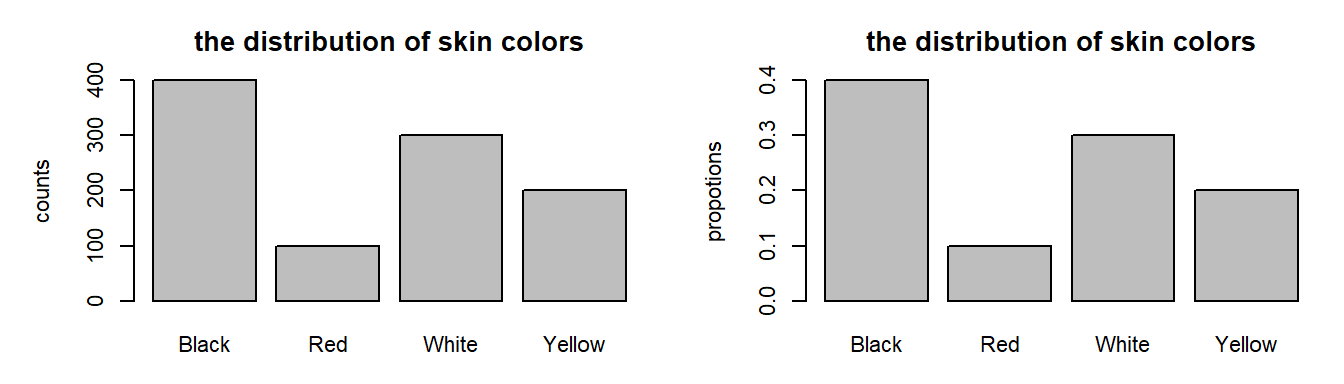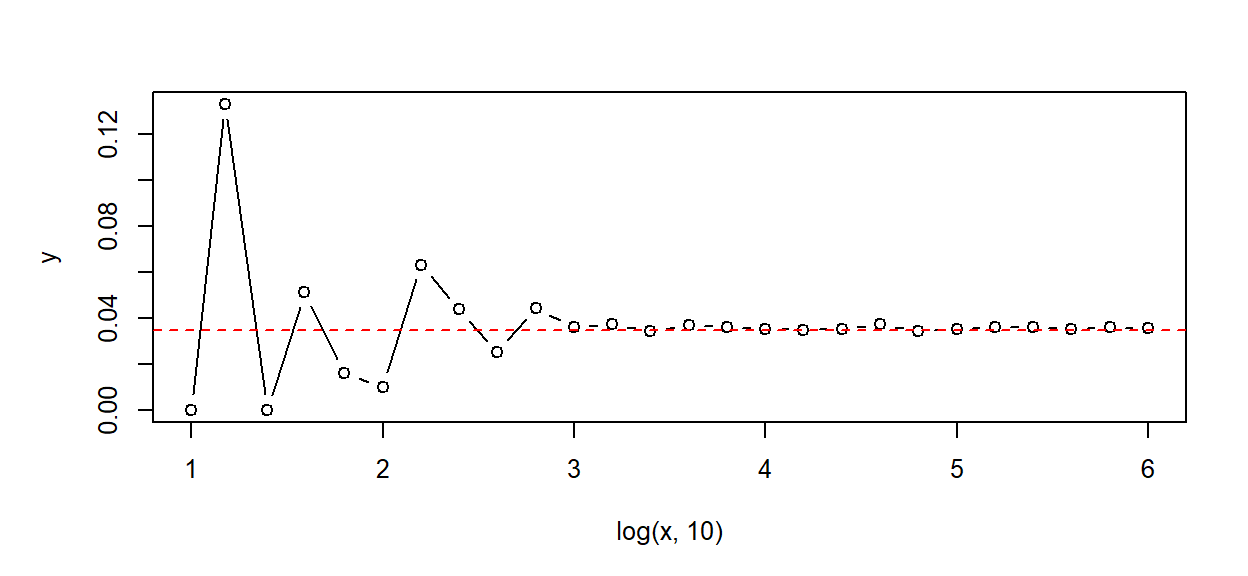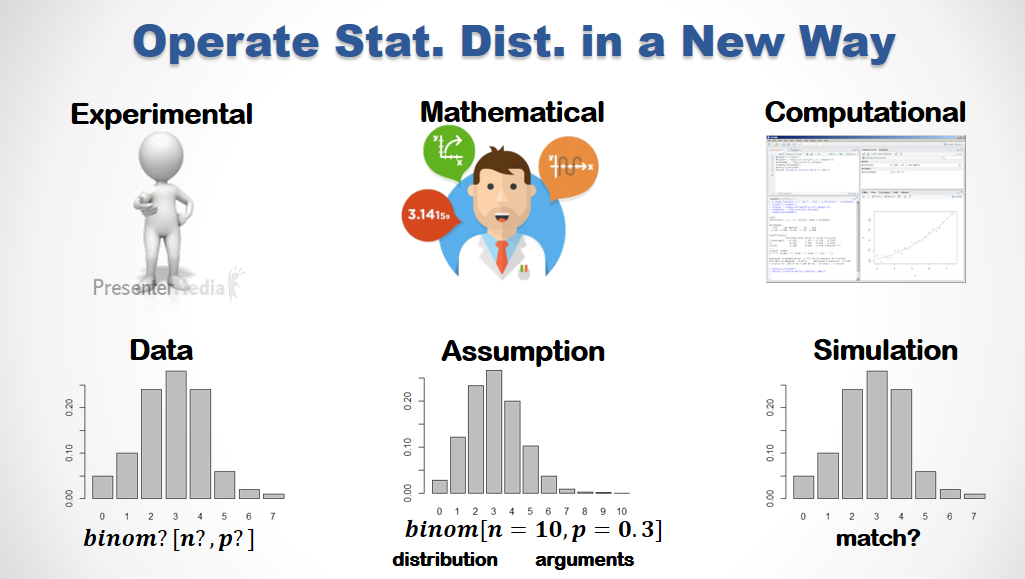👨‍🏫 KEY POINTS :

🔑 A data frame column is an attribute of interest. In terms of statistics, we call it a variable.

🔑 We have a few ways to describe the varying values of a variable.

• Statistics (mean,sd) for numeric and Counts (table) for categorical variables.
• Histograms for continuous/numeric and Bar Plots for discrete/categorical variables.
• Distribution Functions (PDF/CDF) for estimating Probabilities.

🔑 In R we can …

• Represent Variables in Vectors.
• Make Distribution functions directly from data or via simulation.
• Estimate Probabilities with those distribution functions.

pacman::p_load(dplyr)

### 1. Random Draw and Repeatition

Most of the statistics theories were built upon two fundamental assumptions:

• 隨機抽樣 Random Draw
• 無限重複 Infinite Repetition

Mathematically, these hypothetical concepts are somehow difficult to understand. Fortunately, now we can elaborate these conceptual ideas with data in R.

##### 1.1 Random Draw - sample()

The most common random draw function in R is sample()

• sample(x, n) randomly draws n elements from x without replacement
• sample(x, n, replace=T) randomly draw n elements from x with replacement

Randomly draw a number from 1 to 6 …

sample(1:6, 1)
 4

Rut it 10 times, the you’d know what it means by ‘random’.

##### 1.2 Repeated Experiment - replicate()

replicate(n, expr) evaluate the random expr n time

replicate(20, sample(1:6, 1))
  4 1 3 4 6 3 5 5 3 5 3 2 1 6 3 2 5 1 4 3

It returns an vector of 20 integers. Every time you run it, it’ll returns a different result.

### 2. Random Experiment and Variable

##### 2.1 Random Experiment as R Function

In R, we define any Random Experiment as a function that generate random results. It implies that the there are some random elements in the function definition …

exSum3 = function() { sum( sample(1:6, 3, T) ) }

Run it 10 times.

exSum3()
 15

It looks ‘random’, isn’t it.

##### 2.2 Random Variable as the Return Value of Random Experiment

🌻 A Random Variable is defined as the resultant value of a specific Random Experiment.

Because an random experiments return random values, their corresponding random variables varies randomly.

Let’s define a random experiment that returns the maximum value of three fair dices.

exMax3 = function() { max( sample(1:6, 3, T) ) }

When we repeat an random experiment N time, we will have a resultant vector of length N. This resultant vector is a random sample of the corresponding random variable.

If we define a random variable rvMax3 on the random experiment reMax3, we can take a 20-point random sample of rvMax3 by …

rvMax3.20 = replicate(20, exMax3())

🚴 EXERCISE :

👉 Define a random experiment that randomly draw 3 numbers out of 1:10 and returns the minimum.

# Hint: should the replace argument in sample() be TRUE or FALSE?
#
#

👉 Take a random sample of size 100 form the corresponding random variable.

#
#

👉 What is the best way to present this sample to you boss?

#
#

### 3. The Concept of Distribution

Distribution := a formula that describes how the values of a variable spread over a domain.

There are 3 types of distribution:

• Dist. of Real (Empirical) Data observed from the field
• Dist. of Simulated Data generated by some programs
• Dist. of Random Variables are Conceptual and Theoretical
##### 3.1 the distribution of real/simulated data

Supposed that we have a data vector skin that represents the skin colors of a population of 1,000 people.

skin = c(rep('Black',400),rep('White',300),rep('Yellow',200),rep('Red',100))

The distribution of skin can be represented as a numeric table of counts or proportions …

rbind(
counts = table(skin),
factions = table(skin) %>% prop.table
)
         Black   Red White Yellow
counts   400.0 100.0 300.0  200.0
factions   0.4   0.1   0.3    0.2

or we can visualize the distribution in two ways

• in frequency (counts)
• in proportion (fractions)
par(mar=c(3,5,3,1),mfrow=c(1,2),cex=0.7)
table(skin) %>% barplot(main="the distribution of skin colors", ylab="counts")
table(skin) %>% prop.table %>% barplot(main="the distribution of skin colors", ylab="propotions")🌷 Quite often we’d map the proportions in the distribution to the probabilities of their corresponding events. However, this mapping should be conducted carefully, as will be explained below.

##### 3.2 the distribution of random variable

We can define a random variable rvSkin on the random experiment exSkin which randomly draws a data point from skin.

exSkin = function() {sample(skin, 1)}

To take a sample of size 100, we repeat the experiment 100 times.

rvSkin100 = replicate(100, exSkin())

The distribution of this sample is

table(rvSkin100) %>% prop.table 
rvSkin100
Black    Red  White Yellow
0.40   0.05   0.32   0.23 

Is the distribution of the sample exactly the same as that of the data vector skin?

sapply(2:6, function(i) table(replicate(10^i, exSkin())) ) %>% prop.table(2)
       [,1]  [,2]   [,3]    [,4]    [,5]
Black  0.40 0.402 0.3992 0.40095 0.39997
Red    0.06 0.107 0.0953 0.10001 0.10027
White  0.30 0.296 0.3047 0.29985 0.29945
Yellow 0.24 0.195 0.2008 0.19919 0.20031

As the size of the sample increased, the distribution of the sample become closer to that of the data. But it does not meet the exact numbers even when we take a sample of 1 million (10^6).

### 4. The Connection between Distribution and Probability

Theoretically, the sample distribution will asymptotically be equal to the data distribution when the sample size approaches infinity. This is exactly the underlying logic that we can map the distribution of empirical data to the probabilities of the corresponding events.

🔑 KEY POINTS :

• Practically, we can define the probability of an event as the ratio of its occurrence under a very large number of repetitions.
• Probability in not a real thing. It is a theoretical concept that defines on infinite repetitions of random sampling.
• When we use empirical, theoretical or inferential distribution to estimate the probabilities of events, we should remember that since the premises of perfect randomness and infinity never hold, our estimations are always subject to some level of error.

### 5. Simulating for the Expected Value

The Expected Value bears a similar asymptotic implication. Expected values used to be derived from assumptions and deduction mathematically. For common cases, there are a lot of well defined math formula. For example, the combined outcomes of a series of independent events of pre-defined probabilities is named Multinational Distribution. The probability of an Multinational event take the form of …

$p = \frac{n!}{k_1!\,k_2\,...\,k_i!} \; \prod p_i^{k_1} \\ \frac{10!}{4!\,3!\,2!\, 1!} (.4)^4 \, (.3)^3 \, (.2)^2 \, (.1)^1 \; \simeq \; 0.034836$

Randomly drawing 10 points from skin, the probability that we have 4 blacks, 3 whites, 2 yellows and 1 red is less than 3.5%. It’s hard to do the math or remember the formula though.

Fortunately, in R we can simulate the experiment and let the computer estimates the expected value for us. The asymptotic property implies that our estimation would approach the true value when the number of repetitions is large enough. The questions is - how large is enough?

Let’s define a function Simulated.Prob(k) that repeats the experiment sample(skin,10) k times, and return the proportions that the outcome of the experiment match the distribution of our data.

# convert skin in to a factor for faster execution
sf = factor(skin, c("Black","White","Yellow","Red"))
# prepare for parallel simulation
plan(multisession)
zSimulated.Prob = function(k) future_replicate(
k, as.integer(table(sample(sf, 10))), future.seed=TRUE
) %>% apply(2, identical, 4:1) %>% mean

By increasing k, we approach the ‘infinity’ gradually …

x = as.integer(10^seq(1,6,0.2))
t0 = Sys.time()
y = sapply(x, function(k) { cat(k,"- "); zSimulated.Prob(k) })
10 - 15 - 25 - 39 - 63 - 100 - 158 - 251 - 398 - 630 - 1000 - 1584 - 2511 - 3981 - 6309 - 10000 - 15848 - 25118 - 39810 - 63095 - 100000 - 158489 - 251188 - 398107 - 630957 - 1000000 - 
Sys.time() - t0
Time difference of 1.0802 mins

In a few lines of code we can simulate this somewhat complicated experiment. And the the power of modern computer can execute this simulation 2.7 million times in 1.5 minutes.

par(mfrow=c(1,1), mar=c(5,5,3,2), cex=0.8)
plot(log(x,10), y, type="b")
abline(h=0.0348, col='red', lty=2)See? The result of the simulation converges to the theoretical value when the number of repetition exceeds ~10,000 (10^4).

🌞 TAKE AWAYS :

🌻 Modern business is full of complicated cases that are never covered in Statistics textbook.

🌻 When it is too hard to do the math, computer simulation may help to make good enough estimation in reasonable time

🌻 Mastering R so you can simulate whatever you can specify !!!